Get instant live expert help with Excel or Google Sheets“My Excelchat expert helped me in less than 20 minutes, saving me what would have been 5 hours of work!”

#### Post your problem and you’ll get Expert help in seconds.

Your message must be at least 40 characters
Our professional Expert are available now. Your privacy is guaranteed.

# How to Get the nth Match with VLOOKUP in Excel

We can get the nth match of a data with the VLOOKUP function. This can help us to organize data so that if we are looking for a value, it will be displayed. The steps below will walk through the process.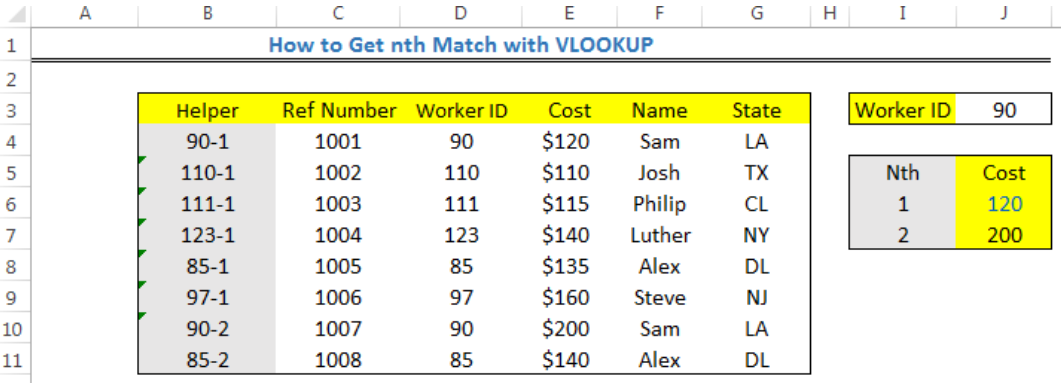Figure 1: Result of the nth match with VLOOKUP

## General formula

`=VLOOKUP(id_formula,table,4,0)`

## Formula

`=VLOOKUP(id&"-"&I6,data,4,0)`

## Setting up The Data

We will input the data as shown in figure 2 into the respective columns and cells.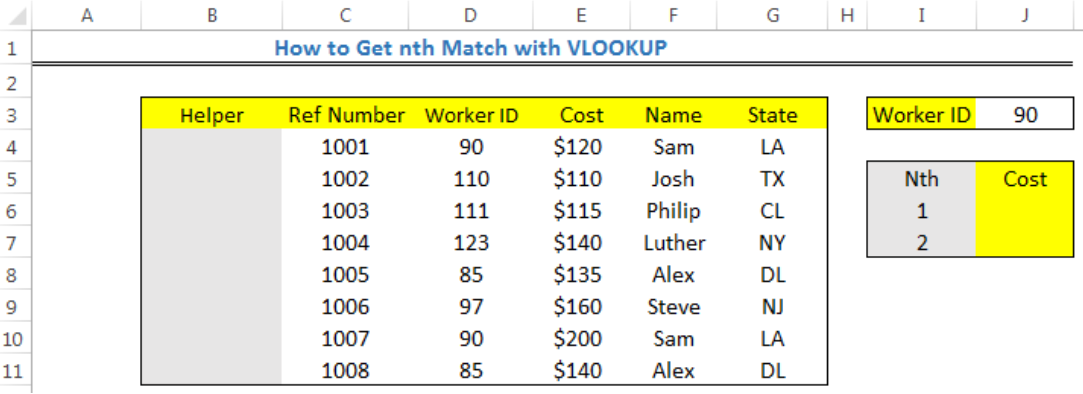Figure 2: Setting up the data to get the nth match with VLOOKUP

## Setting up the Helper Column

• We will click on Cell B4
• We will insert the formula below into Cell B4

`=D4&"-"&COUNTIF(\$D\$4:D4,D4)`

• We will click on enter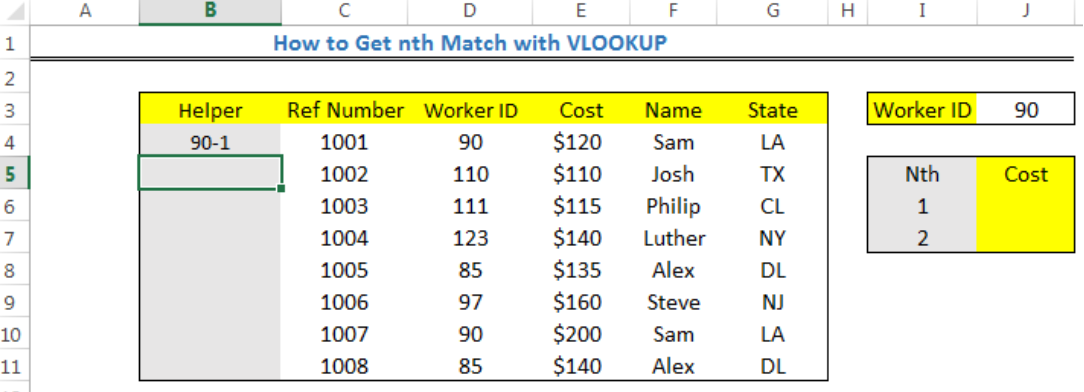Figure 3: Setting up the Helper Column to get the nth match with VLOOKUP

• We will use the drop-down feature to complete the values for the helper column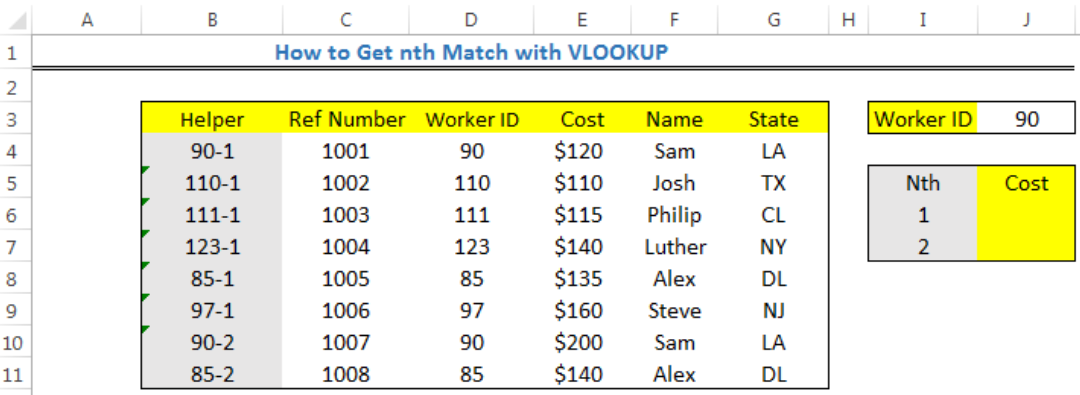Figure 4: Setting up the Helper Column to get the nth match with VLOOKUP

## Finding the Nth Match

• We will click on Cell J6
• We will insert the formula below into Cell J6

`=VLOOKUP(J3&"-"&I6,B4:G11,4,0)`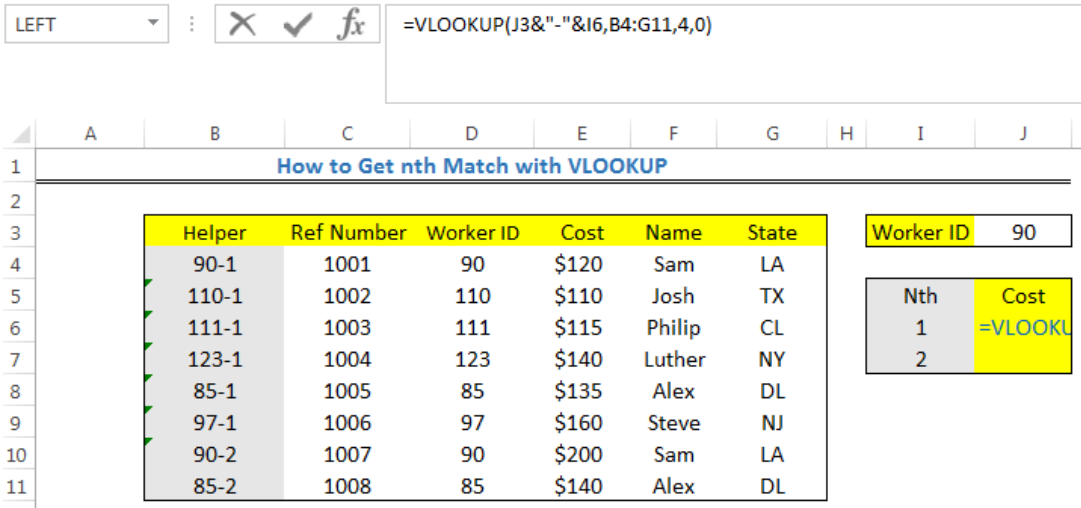Figure 5: Finding the nth match with VLOOKUP

• We will click on enter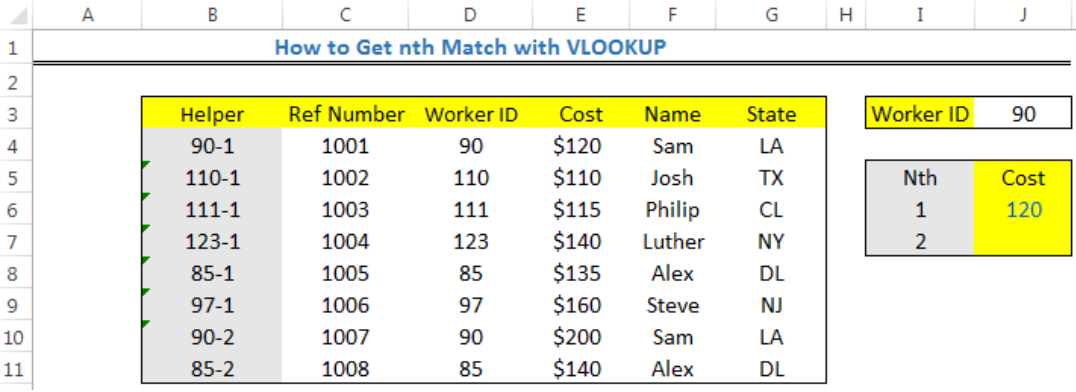Figure 6: Finding the nth match with VLOOKUP

• We will input the formula below into Cell J7 and repeat the steps

`=VLOOKUP(J3&"-"&I7,B4:G11,4,0)`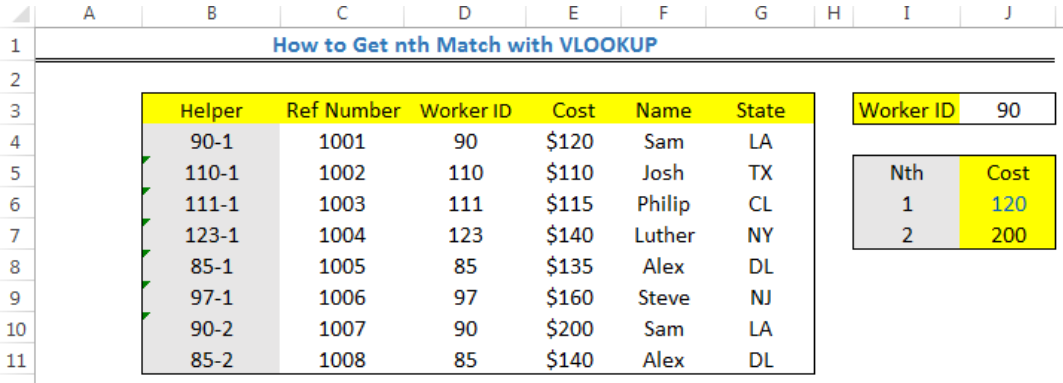Figure 7: Result of the nth match with VLOOKUP

## Explanation

• Helper Column

We use the helper column to construct an identity that includes the count. The formula we used to create the helper column from the steps builds a unique lookup value based on the worker ID and a counter that detects the number of times the unique ID has been displayed in the data table.

• COUNTIF Function

The COUNTIF function is used to generate a running count of the ID in the data. For instance, Cell B4 and Cell B10 is an example of a running count.

• VLOOKUP Function

VLOOKUP is used for getting values from the table, taking into cognizance the “nth” occurrence.

## Instant Connection to an Expert through our Excelchat Service

Most of the time, the problem you will need to solve will be more complex than a simple application of a formula or function. If you want to save hours of research and frustration, try our live Excelchat service! Our Excel Experts are available 24/7 to answer any Excel question you may have. We guarantee a connection within 30 seconds and a customized solution within 20 minutes.

Are you still looking for help with the VLOOKUP function? View our comprehensive round-up of VLOOKUP function tutorials here.

### Did this post not answer your question? Get a solution from connecting with the expert.Another blog reader asked this question today on Excelchat:
Solution examplesI have a pivot table in which per order (on the rows) stands how much products they ordered per size (on the columns). I want to determine which combinations of quantities of sizes people order. And I want to count these combinations.
Solved by E. W. in 60 minsI have 500 numbers in column A with aproximatly 15digits. In column B I have 5 numbers with 6 digits Can a find a formula wich can transfer all numbers from A column wich start with some 6 digitsa from B column
Solved by V. L. in 32 minsI have a sheet with 3 columns. First column is a code for records in column B (has 563 records). What I need is a formula to show me if what is in column C (has 4400 records) is in column B and if it is to take the code for that record. Example: Column A(code corresponding to column B): 12, 14, 15, 19 Column B(names): asd, adf, ade, aqw Column C(names): akd, adf, ade, anb, wgs I need something that would say, if record in column C (for example adf) is matching record in column B (adf) return the code from column A (the code corresponding to that record in column B),if not return blank.
Solved by C. J. in 8 minsIf the data in A matches the data in C, then I need the data in E to be placed in B
Solved by X. W. in 40 minsI have a workbook with 2 sheets. First sheet has column A which is a device name, then columns B - infinity that have numeric "tags" for that device. Each device can have one or 20 "tags", tags are all numerical. Worksheet 2 has column A which is numeric value and B which is a human readable value of what the tag is. Question is, how do I replace all the tag numbers in worksheet 1 with the actual human readable values is sheet 2?
Solved by T. H. in 16 mins## Subscribe to Excelchat.coAnother blog reader asked this question today on Excelchat: